# Proof Of Concept

Verities never lie.

# Entire circuits test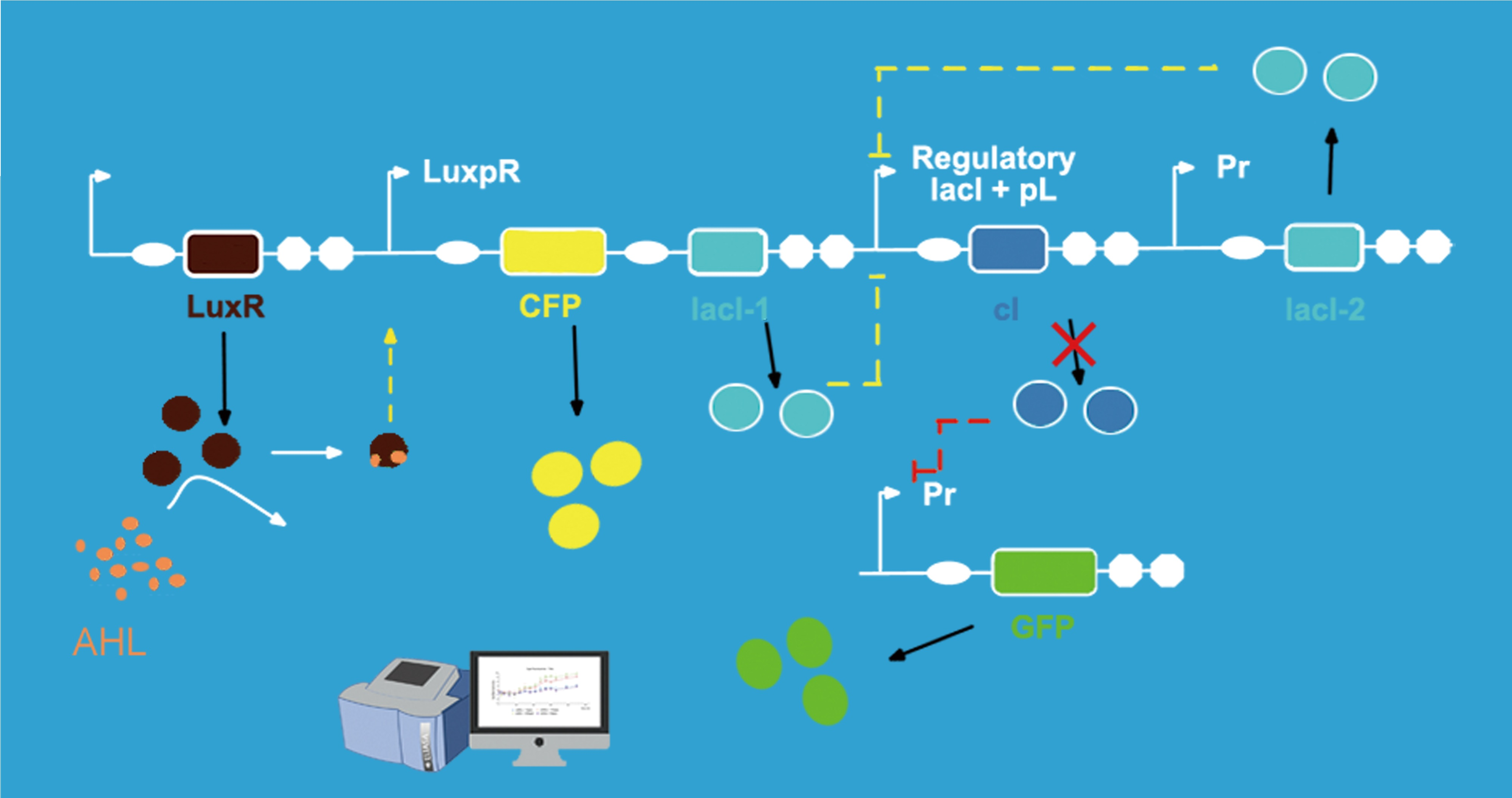In our experiment, for testing our circuit more conveniently, we use CFP to replace the responder and use GFP to replace lysis. Because the fluorescence intensity is easier to be measured. Also, the intensity of the fluorescence can stand for the intensity of the corresponding genes' expression.

When the concentration of AHL is at low concentration, AHL cannot be detected by the quorum sensing system. And as a consequence, there is no enough inhibitor lacI to repress promoter Plac. So the cI gene is expressing at the wild-type levels. While lacI-2 and GFP are repressed since the CI protein is at high concentration. When AHL around the engineered bacteria reached a certain concentration or a threshold value, AHL could be detected by the sensing molecules Lux R. Then, Lux R interacted with AHL and form activating molecules to activate promoter Lux pR. CFP and lacI-1 express, and CFP protein could be detected by fluorescence measurement. And the lacI-1's product-lacR protein could inhibit the expression of cI gene. As a result, lacI-2 and GFP expressed. The lacI-2's product could further repress cI gene's expression, which was a negative feedback system. GFP could be measured by fluorescence detection to represent the level of lysis gene's expression. The time of initial expression of CFP and GFP were different. As a result, the interval of time could represent the delay time which the switch give for responder expression.

As we can see, quorum sensing system could be induced by AHL. As for our gene circuits, when AHL raised to its threshold value and was detected by the sensor part, the promoter Lux PR can be activated, CFP and GFP are expressed. In order to find out the relationship, we decided to make an AHL gradient induction for our gene circuits.

During our experiment, we first constructed our gene circuit successfully, then we transformed the plasmids into chemical competent cell (DH5α). And then our engineering bacteria were divided into four groups: experimental group 1, 10 and 20 (c(AHL) = 1 ng/μL, 10 ng/μL, and 20 ng/μL) and blank control group. Then we measured OD600 of the E.coli and the fluorescence intensity of CFP and GFP for 900 minutes. The OD600 showed the growth trend of the engineering bacteria. And the fluorescence intensity of each group showed the relationship between AHL and the expression of our circuits.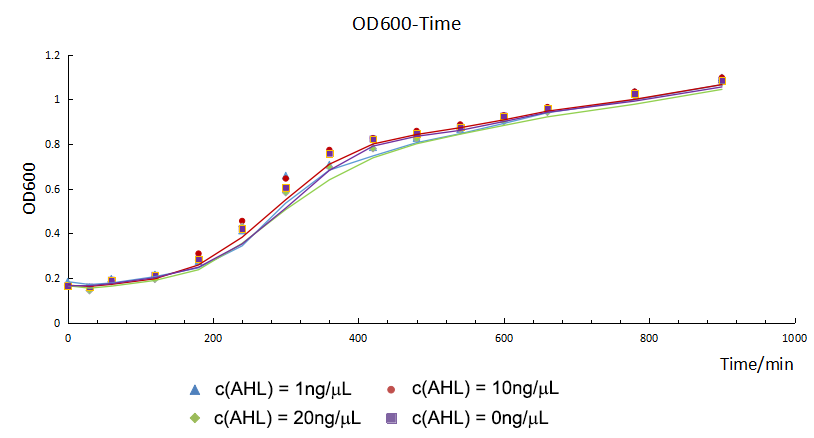Figure 1.1 The OD600 of versus time of experimental group 1, 10, 20 and control

From figure 1.1, we found that the growth curves of each group don't show much difference. It suggested that they had the same growth trend, so the difference of fluorescence intensity was mainly from the expression of circuits or other factors.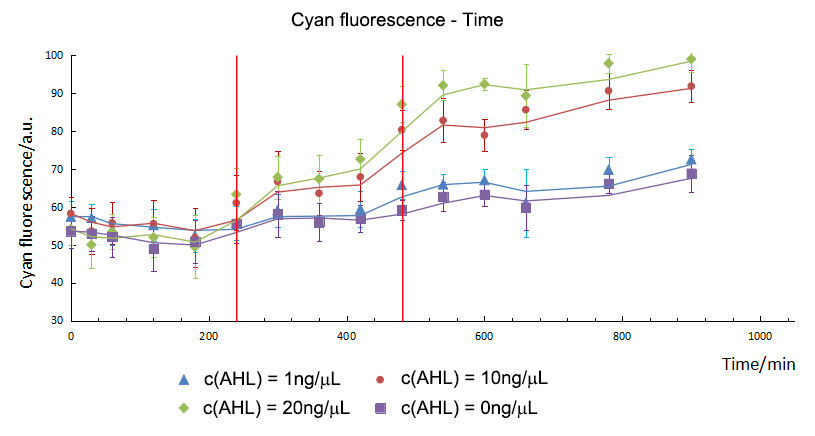Figure 1.2The average cyan fluorescence intensity versus time of experimental group 1, 10, 20 and control.

So we did experiments at the same condition except the concentration of AHL. As showed in figure 1.2, with the increase of the AHL, the expression of the CFP showed an upper trend. In addition, by means of independent t-test, we compared the fluorescence intensity between the group 1, 10, 20 and control. We found the initial expression time of CFP was different. For group 10 and 20, CFP expressed initially and could be detected in the 240th minute. However, for group 1, CFP started to express in the 480th minute.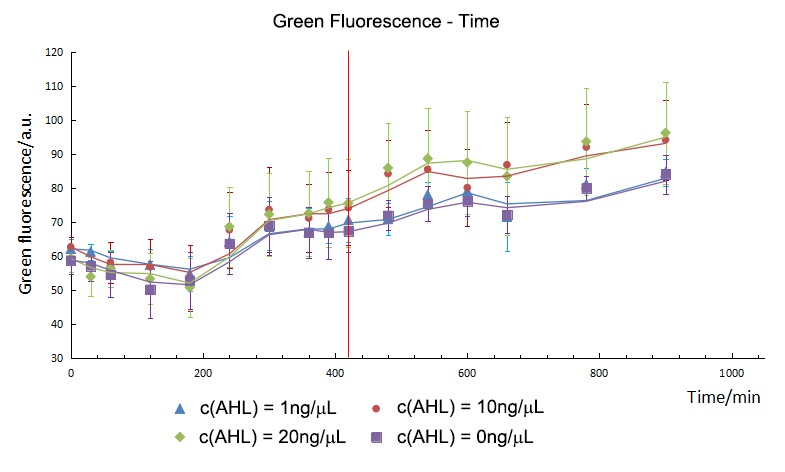Figure 1.3 The average green fluorescence intensity versus time of experimental group 1, 10, 20 and control.

T-test showed that AHL didn't influence the expression of GFP directly. Group 10 and 20 expressed initially in the 420th minute and green fluorescence intensity had no significant difference in expression level. However, as for group 1, green fluorescence intensity didn’t show much difference comparing with control group, which meant that 1 ng/μL of AHL was not enough to activated the sensor successfully. As a result, the repression on the Plac cannot be released, and the expression of gfp still stayed in a low level. So the sensor part is an specific and smart device.

### 2. The Delayed Effect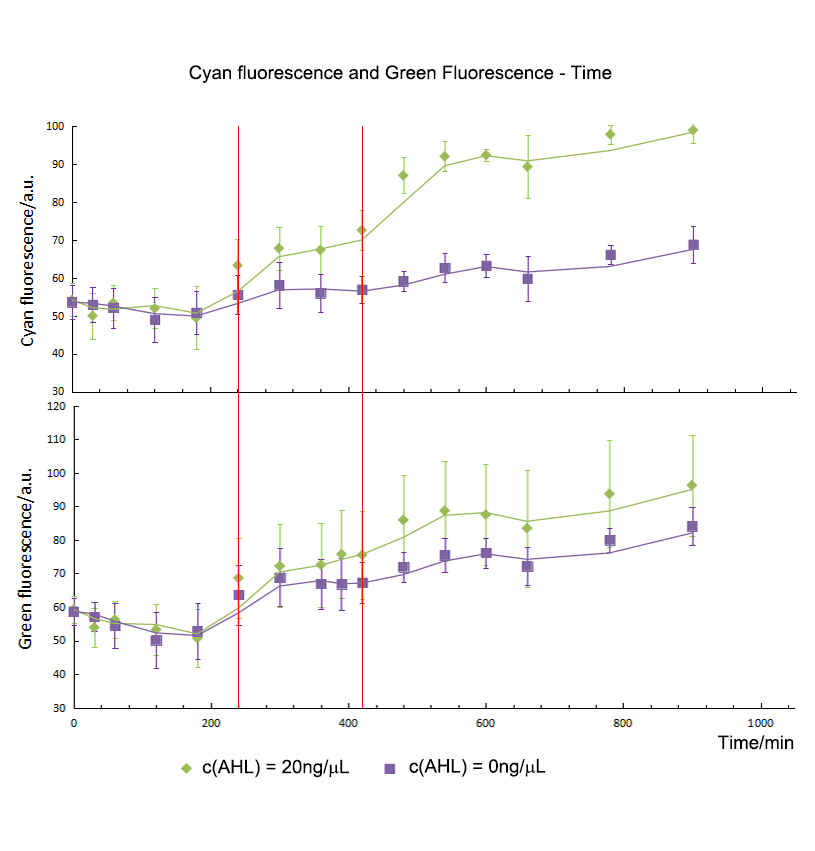Figure 1.4 The average cyan and green fluorescence intensity versus time of experimental group 20 and control.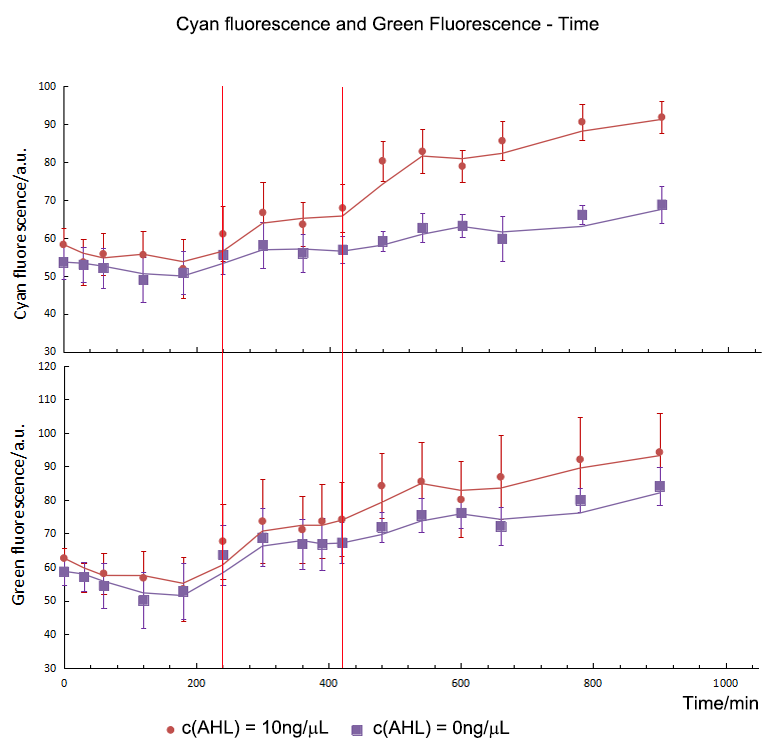Figure 1.5 The average cyan and green fluorescence intensity versus time of experimental group 10 and control.

As mentioned above, we did analyze the fluorescence intensity data by means of t-test to show that if there was a significant variation between experiment groups and control. And then, we get the initial expression time of CFP/ GFP in experiment groups and control. For group 10 and 20, the initial expression time of CFP was the 240th minute and GFP was detected at the 420th minute. From the results, we could draw the conclusion that the expression time of GFP was delayed to 180mins post CFP, which was the same as our expectation. So the Sensor and switch expressed as expected and match with each other very well.

# Responder test

Against the backdrop of today's widely spread of drug-resistant bacteria, small regulatory RNA (sRNA) offers a new clinical therapy approach to repress the target drug-resistant genes and then it can enhance the effect of antibiotic in the clinical. Here we designed synthetic sRNA to target the antibiotic resistance gene chloramphenicol acetyltransferase (CAT) to generate representative data and to quantify knockdown effectiveness.

We designed and synthetized RNA silencing cassettes (Table 1) and constructed it into pSB1AC3, an official plasmid which contained ampicillin resistance gene and chloramphenicol resistance gene. Also, we used pSB1AC3+mRFP as control.Figure 2.1 The diagram of synthetized RNA silencing cassettes and control.

Part Sequence
pR promoter TAACACCGTGCGTGTTGACTATTTTACCTCTGGCGGTGATAATGGTTGC
GUIDE sequence ATATCCAGTGATTTTTTTCTCCAT
Hfq binding domain TTTCTGTTGGGCCATTGCATTGCCACTGATTTTCCAACATATAAAAAGA
CAAGCCCGAACAGTCGTCCGGGCTTTTTTTCTCGAG
T1/TE terminator TTTCGTTTTATCTGTTTTTGTCGGTGAACGCTCTCTACTAGAGTCACACT
GGCTCACCTTCGGGTGGGCCTTTCTGCGTTTATA

Table 1 The sequence of synthetized RNA silencing cassettes

Firstly, we designed and synthetized RNA silencing cassettes and construct it into pSB1AC3, an official plasmid which contains amp and cm resistance gene. Also, we used pSB1AC3+mRFP as control. Then we transfer the plasmids into chemical competent cell (DH5α) and add chloramphenicol of 50μg/ml to detect the knockdown effectiveness through the phenotype of survival index. We continuously measured the OD600 through the Bioscreen.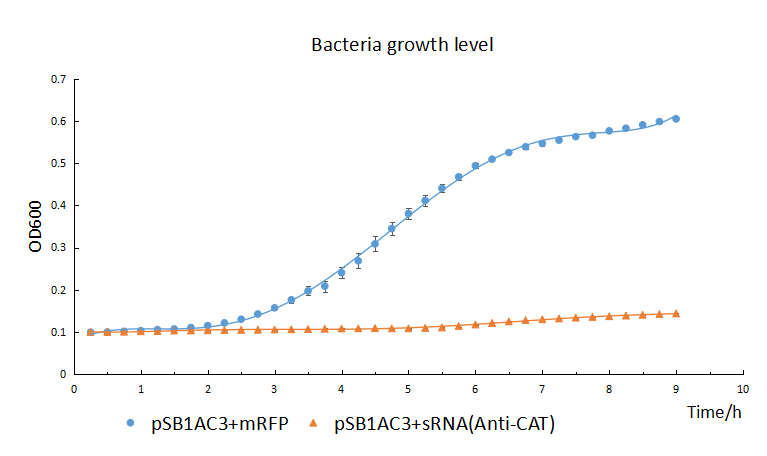Figure 2.2 The growth curves of bacteria with the sRNA cassettes or mRFP on the chloramphenicol of 50ng/μL.

The growth of chemical competent cells(DH5α) with pSB1AC3+sRNA is significant repressed by the chloramphenicol (50μg/mL) compared with the control with pSB1AC3+mRFP (The blue line). It indicated the sRNA’s highly knockdown effectiveness.

From the result, we can found the OD600 of control group shows S-type growth trend. But the experimental group shows a low level all the time. It suggests the growth of the bacteria which contain the sRNA cassettes significantly repressed by the chloramphenicol, which indicates the sRNA’s highly knockdown effectiveness.

# Model

Abstract: E. coli has been modified in order to produce small interfering RNA molecules (siRNA) to attack the resistance genes that become available for nearby bacteria, when a resistant strain goes through lysis. However, a regulation system should be applied so the productive cells do not become a problem. A circuit has been implemented, this circuit regulates the transcription of said siRNAs among the production of toxins that could lead to death of any nearby cell, including the siRNA producers themselves. Taking in consideration all these elements a mathematical model using differential equations has been designed to approach the number of viable cells within the toxic environment once the circuit has been activated.

Keywords: siRNA, toxin, mRNA, transcription, translation, exponential

### Rate of production of toxin mRNA and toxin molecules

The toxins are produced through a gene in the circuit, so the production of toxin depending on the translation of the mRNA produces, which also depends on transcription variables. A differential equation was taken in consideration in order to measure the mRNA rate production.

$$\frac{dr}{dt} = K - D_{r}*r$$

$$K$$ represents the transcription rate, which is a constant parameter, in molecules of RNA per second, $$D_{r}$$ is the degradation rate expressed in units per seconds as it depends on how much mRNA is actually present on the cell, expressed by the variable "$$r$$" in units of molecules of mRNA produced.

$$r = \frac{K}{D_{r}}+Ce^{-D_{r}t}$$

Afterwards, the original mRNA production equation was obtained through the integration of the differential equation by linear integration.

Establishing a new condition $$r(0)=R_{0}$$ then we can complete the system. Substituting we obtain:

$$R_{0} = \frac{K}{D_{r}}+C \rightarrow C = R_{0}-\frac{K}{D_{r}}$$

We finally obtain the equation for mRNA synthesis:

$$r(t)= \frac{K}{D_{r}}+(R_{0}-\frac{K}{D_{r}})e^{-D_{r}t}$$

To simplify the model we assume that each mRNA molecule will be transcribed in order to produce one molecule of protein. This means that each molecule of mRNA produced is equivalent to one molecule of active toxin that in certain concentration can kill bacteria. We assume a fast degradation of mRNA inside the system and almost no significant dispersion of the molecules, so this ratio can be respected.

### Productive cells inside the system

The number of E. coli cells within the system can not be considered as a constant, neither as a linear function due to the exponential nature of cellular division. Given normal growth of the cell culture, it is possible to describe the number of E. coli cells as a function of time. Nevertheless, the E. coli concerning the model produces toxins that alter the number of E. coli cells itself, also there is a natural decrease in the number of bacteria caused by cellular death, resulting in the addition of new terms to the traditional equation. An equation considering all the previous factors is shown below.

$$C(t)=C_{0}e^{Gt}(1-D)-M*C$$
$$C(t)=C_{0}e^{Gt}(1-D)-M*C_{0}e^{Gt}(1-D)$$
$$C(t)=C_{0}e^{Gt}(1-D)(1-M)$$

Where:
$$C_{0}$$: Initial population [=] cells
$$C$$: Total productive cells [=] cells
$$D$$: Cell fraction ( $$C_{0}e^{Gt}(1-D)$$ ) ​that dies naturally [=] adimensional < 1
$$G$$: Rate of cells that are born [=] 1/second
$$M$$: Fraction of viable cells ( $$C_{0}e^{Gt}(1-D)$$ ) that lyse due to toxins [=] adimensional < 1
$$D$$ and $$M$$ are not related, therefore they are independent. It is also important to notice that we assumed that the number of toxins equals the number of mRNAs transcribed, so our previous defined function $$r(t)$$ can help us to determine the constant $$M$$.

$$r(t) < X$$: no cell death, $$M = 0$$

$$r(t) \geqslant X$$: cell death occurs, $$M=\frac{T r(t)}{(1-D)C_{0}e^{Gt}}$$ < 1

where $$X$$ is the critical toxin production rate. Also in order to determine the $$M$$ term we need other variables.

$$M=\frac{T r(t)}{(1-D)C_{0}e^{Gt}}$$, where:

$$r(t)$$ = toxins produced in time $$t$$ [=] (molecule of toxin)
$$T$$ = cells that die by unit of toxin [=] (cells / molecule of toxin)
$$M$$ = dead cells / total viable cells [=] adimensional

for $$r(t) < X$$: $$C(t) = C_{0}e^{Gt}(1 − D)$$ (3)

for $$r(t) \geqslant X$$: $$C(t)= C_{0}e^{Gt}(1-D)(1-\frac{T r(t)}{(1-D)C_{0e^{Gt}}})$$ (4) then

$$C(t) = C_{0}e^{Gt}(1 − D) − T r(t)$$ (5)

The coupled equations yield the following final modelling:

$$C(t)=C_{0}e^{Gt}(1-D)- T[\frac{K}{D_{r}}+(R_{0}-\frac{K}{D_{r}})e^{-D_{r}t}]$$

# Discussion

From the experiment results, we found that the entire gene circuits can express well. Sensor was a specific and smart device, which could detect the quorum sensing molecules AHL and activated the following gene circuits when AHL reached threshold value and stayed “off” state under the threshold value. Switch was a perfect device, which delay the expression of lysis (represented by gfp) so that responder (represented by cfp) had enough time to express. Also, responder test showed that sRNA is a dramatically weapon with high knockdown effectiveness. So if we connected these perfect parts into a synthetic biological device and transformed it into chemical competent cell (DH5α), we can get powerful bacterial soldier to fight and beat antibiotic resistant bacteria.

# Reference

 Bernheim, A. G., Libis, V. K., Lindner, A. B. & Wintermute, E. H. Phage-mediated Delivery of Targeted sRNA Constructs to Knock Down Gene Expression in E.coli. J. Vis. Exp. 109, 1–10(2016).
 Kobayashi, H., Kærn, M. & Araki, M. et al. Programmable cells: Interfacing natural and engineered gene networks. PNAS 101, 8414-8419(2004).

Name: XMU-China School: Xiamen University

Address: Xiamen University, No. 422, Siming South Road, Xiamen, Fujian, P. R. China 361005

Name: XMU-China School: Xiamen University

Address: Xiamen University, No. 422, Siming South Road, Xiamen, Fujian, P. R. China 361005